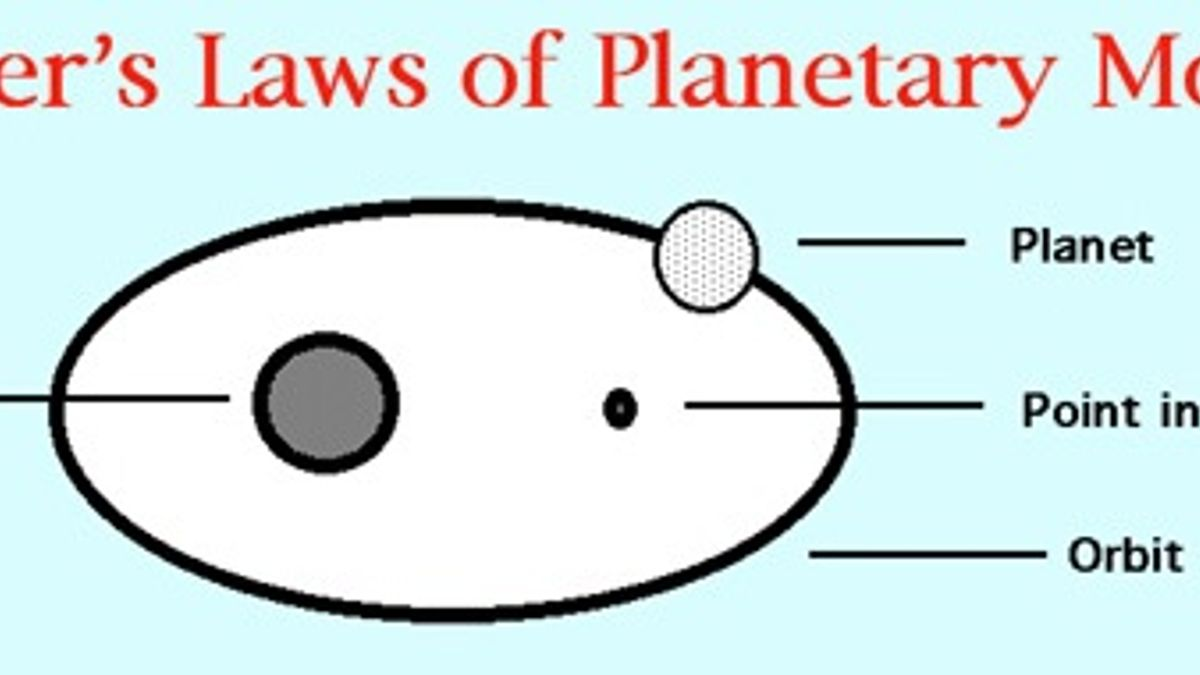# What are Kepler’s laws of Planetary Motion?

Kepler’s planetary law of motion describes the motion of satellites around the planets. Let us study through this article what are Kepler’s three laws of planetary motion with explanation.What is Kepler’s laws of Planetary Motion?

Johannes Kepler was a 16th century astronomer who established three laws which govern the motion of planets around the sun. These are known as Kepler’s laws of planetary motion. These laws describes the motion of satellites like the moon around the planets like the earth.
Kepler’s Laws of Planetary Motion
1. Kepler’s first law: The planets move in elliptical orbits around the sun, with the sun at one of the two foci of the elliptical orbit. This means that the orbit or path of a planet around the sun is an ellipse i.e. an oval-shaped and not an exact circle. An elliptical path has two foci and the sun is at one of the two foci of the elliptical path. This law is important for us as it helps us discover if other stars have planets! We cannot see those planets but if the star wiggles back and forth in a complicated way, it may be because a planet makes it move so.

2. Kepler’s second law: Each planet revolves around the sun in such a way that the line joining the planet to the sun sweeps over equal areas in equal intervals of time. We know that a planet moves around the sun in an elliptical orbit with sun at one of its focus. Now, since the line joining the planet and the sun sweeps over equal areas in equal intervals of time, it means that a planet moves faster when it is closer to the sun and moves slowly when it is farther from the sun.

Source: www. world-builders.org.com

How Gravitational Force holds the Solar System together?
In the above figure a planet P is moving in an elliptical orbit around the sun. When the planet is nearer to the sun at position A, it travels faster and sweeps over an area ABC in time t. On the other hand, when the same planet is farther from the sun at position X, then it moves slowly but sweeps over an equal area XYC in the same time t. Thus the Kepler's second law states that a planet does not move with constant speed around the sun. The speed is greater when the planet is nearer the sun and less when the planet is farther away from the sun. A planet could move around the sun with constant speed only if its orbit were a true circle and not an ellipse.
With the help of second law of Kepler’s we come to know that the Earth is closest to the Sun on 4rth January, when day and night are equal etc.
3. Kepler’s third law:  The cube of the mean distance of a planet from the sun is directly proportional to the square of time it takes to move around the sun.

Source: www.image.slidesharecdn.com
With the help of Kepler’s third law of planetary motion we can show how long does it takes to reach Mars, how long would it take for a spacecraft from earth to reach the Sun, how far from the center of Earth do synchronous satellites orbit, how far does Halley’s comet go etc.
Though Kepler gave the laws of planetary motion but he could not give an explanation about the motion of planets. It was Newton who showed that the cause of motion of planets is the gravitational force which the sun exerts on them. In fact, Newton used the Kepler’s third law of planetary motion to develop the law of universal gravitation.

What is momentum and its importance in everyday life?

Get the latest General Knowledge and Current Affairs from all over India and world for all competitive exams.
खेलें हर किस्म के रोमांच से भरपूर गेम्स सिर्फ़ जागरण प्ले पर